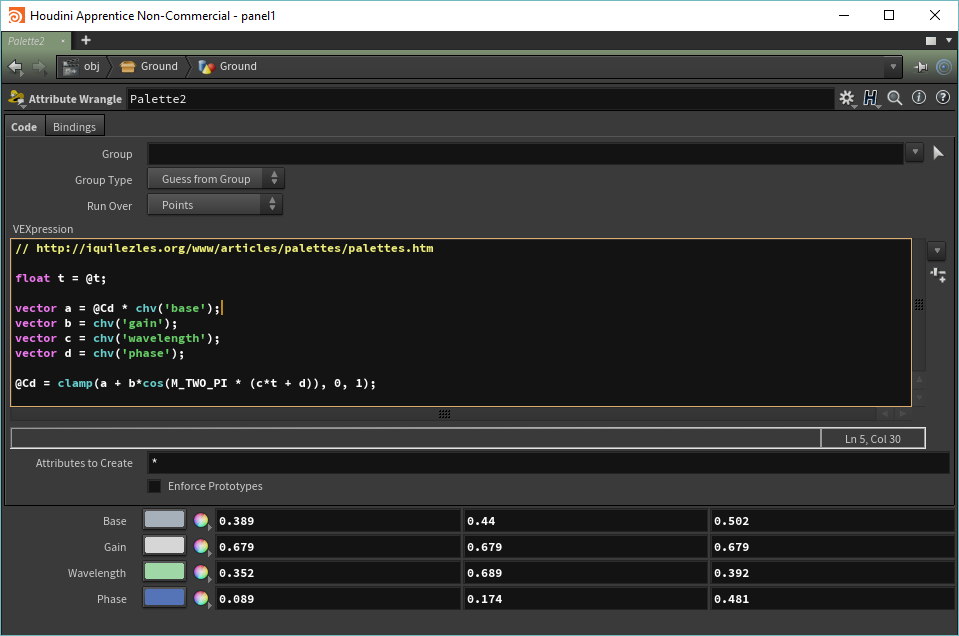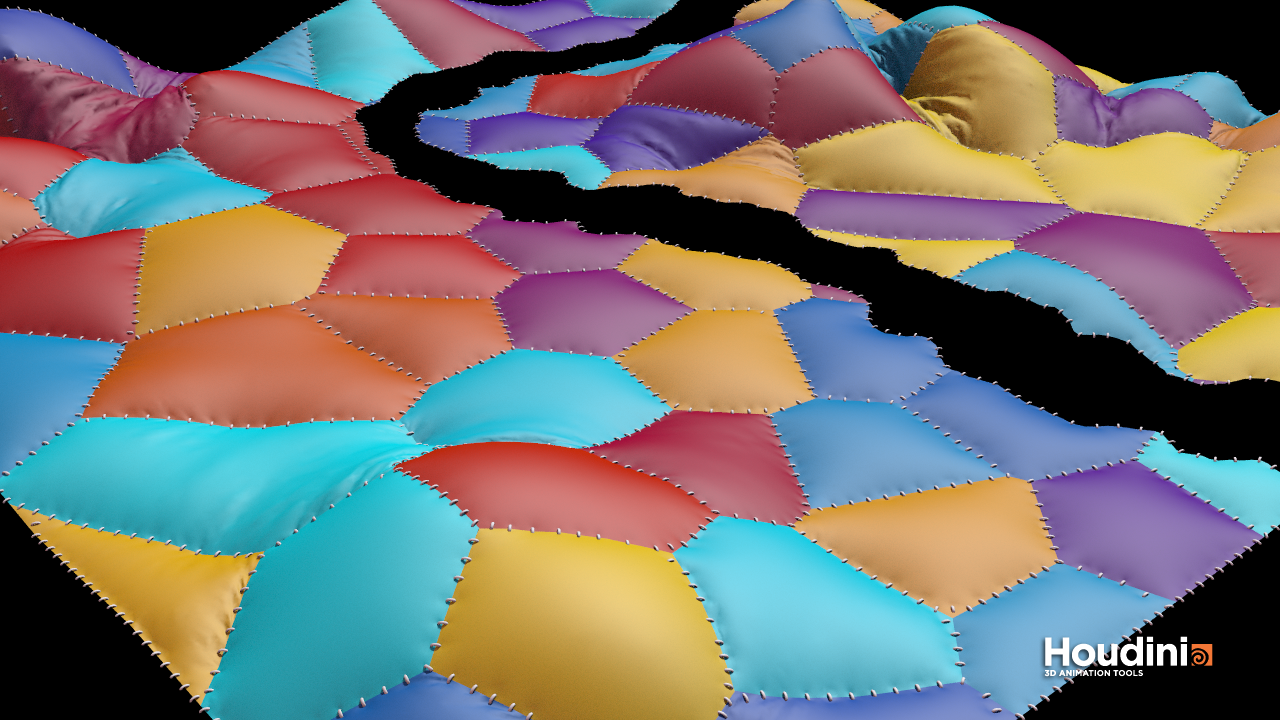Procedural Color Palette

I found this awesome article about Procedural Color Palette and thought that would be very useful for my Houdini projects.

Without further ado, here is the Attribute Wrangle implementing this algorithm:`float t = @t; vector a = @Cd * chv('base');vector b = chv('gain');vector c = chv('wavelength');vector d = chv('phase'); @Cd = clamp(a + b*cos(M_TWO_PI * (c*t + d)), 0, 1);`

In that particular case, I’m using @t point attribute to choose the final color, but obviously something else could be used.

Here is the result: